# HomeEnergy - Pi

Turn a Raspberry Pi into a home energy monitor with inexpensive components.

IntermediateFull instructions provided8 hours8,218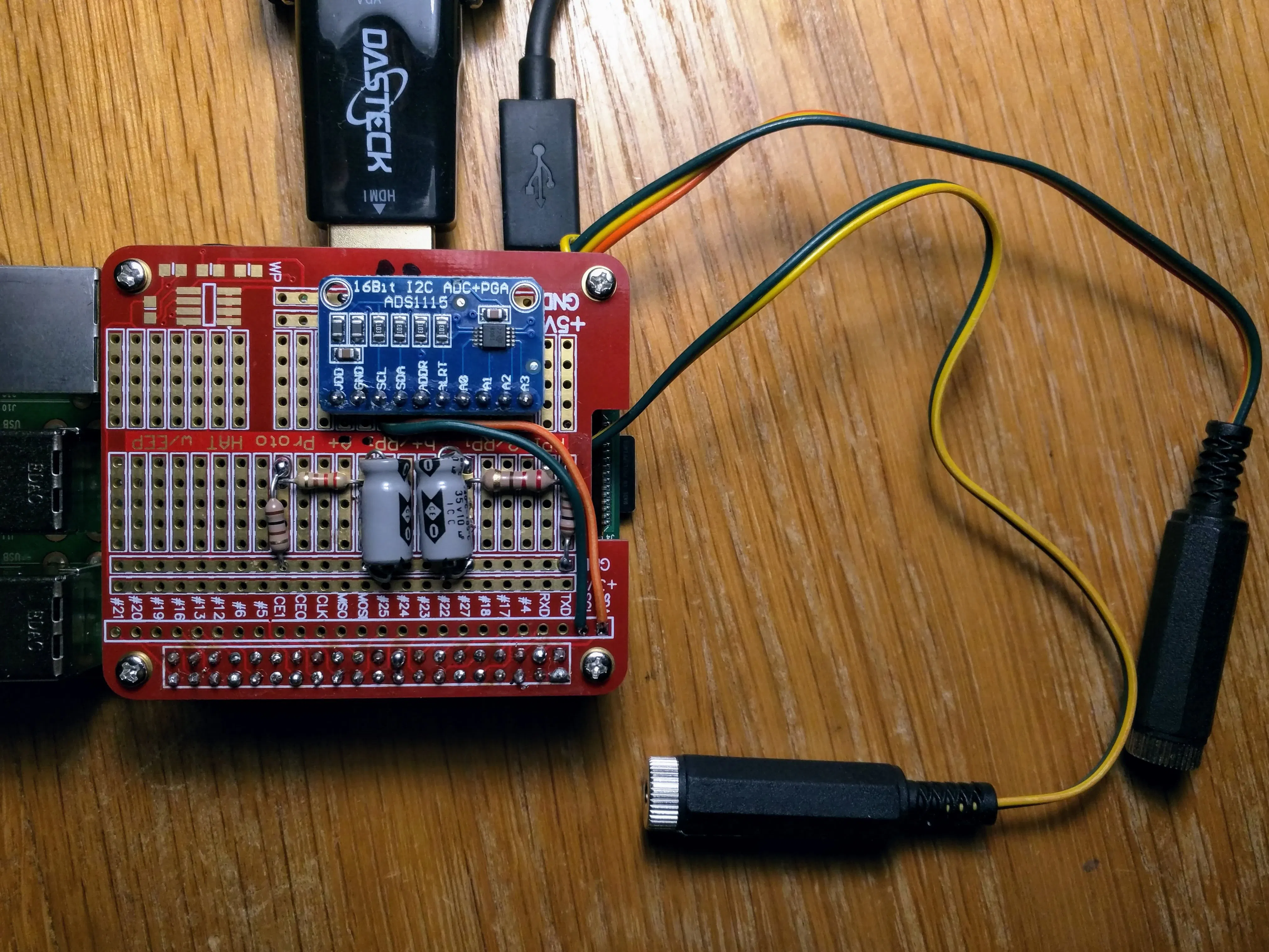## Things used in this project

### Hardware components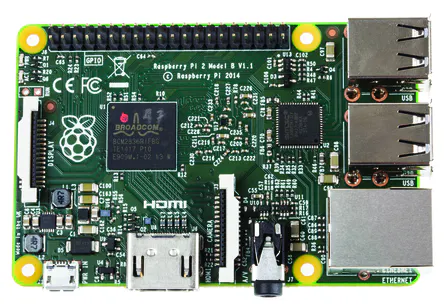Raspberry Pi 2 Model B Newer models should work fine, too
×1
×1
 SCT-013 Inductive Current Sensor One is enough to experiment. To monitor both legs of a typical home power panel, you'll need two of these.
×2
 GPIO Breakout Board
×1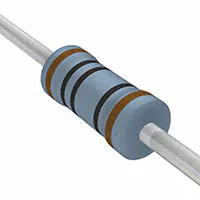Resistor 100 ohm
×1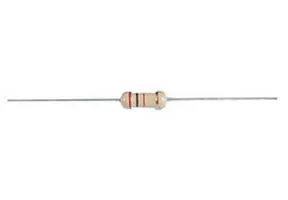Resistor 220 ohm
×2Capacitor 10 µF
×2
 Prototype Hat for Rasberry Pi When everything is working on the breakout board, you can transfer it to this soldered prototype board that fits on top of the PI.
×1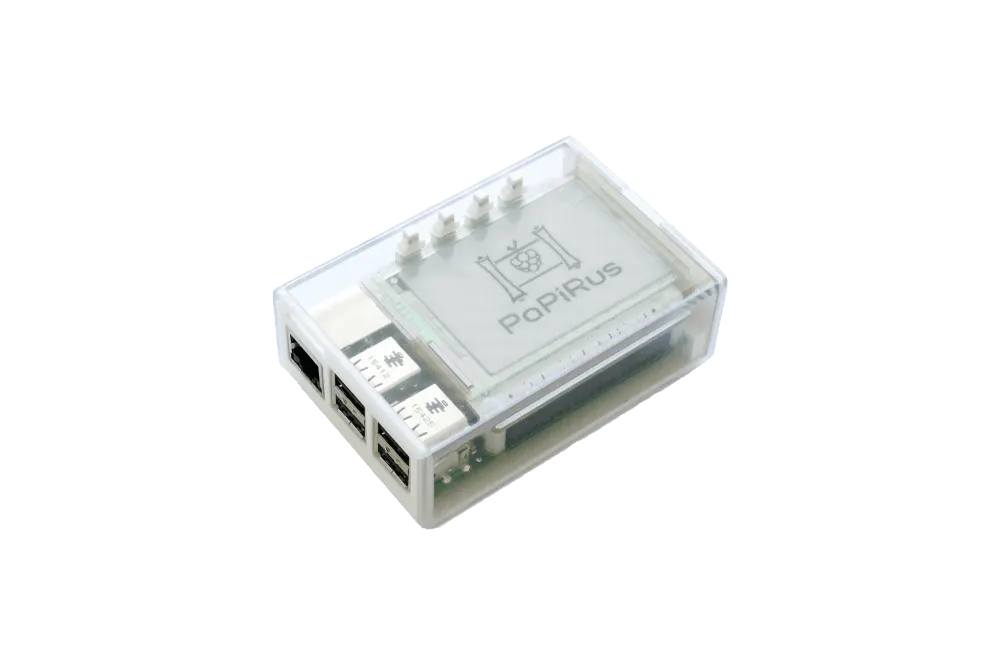Pi Supply PaPiRus HAT Case A case to put the Pi that's large enough to include the hat. I found several others that would work just as well.
×1

### Software apps and online services

 Raspberry Pi Raspian

## Schematics

### Schematic

This circuit diagram shows how the AC current produced by the sensor is turned into a voltage that can be sampled by the analog-to-digital converter.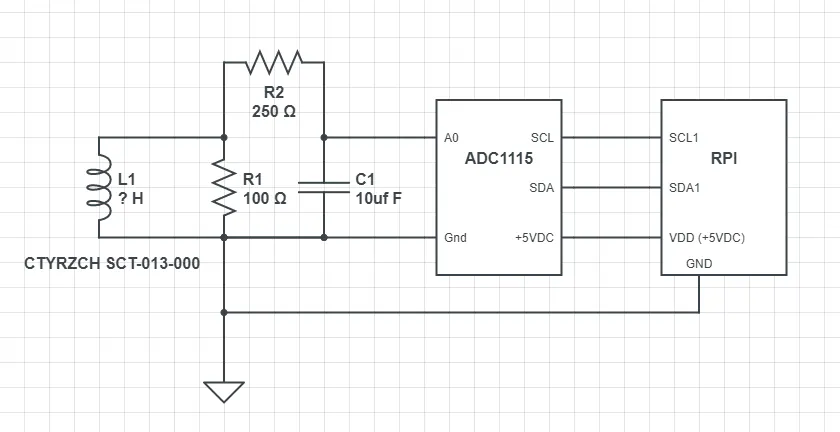## Code

### Sampler.py

Python
This python routine takes a sample from the ADC and saves it to a database table. The code can also be found in the GIT repository.
```# -------------------------------------------------------------------------
#
# Copyright (C) 2018 Michael T. Nigbor
#
#    This program is free software: you can redistribute it and/or modify
#    the Free Software Foundation, either version 3 of the License, or
#    (at your option) any later version.
#
#    This program is distributed in the hope that it will be useful,
#    but WITHOUT ANY WARRANTY; without even the implied warranty of
#    MERCHANTABILITY or FITNESS FOR A PARTICULAR PURPOSE.  See the
#    for more details.
# -------------------------------------------------------------------------
import time
import math
import sqlite3
import datetime
import array
import json

dt = datetime.datetime.now()
print("===== sampler.py starting at ", dt.isoformat())

try:
with open('/home/pi/HomeEnergy/HomeEnergy.json') as json_data:
except IOError as e:
print("Unable to open configuration file:", e)
exit()

GAIN = config["Sampler"]["gain"]

# Polynomial regression coefs to estimate amps
# Based on experiments from 0 to 13 amps
A = config["Sampler"]["A"]
B = config["Sampler"]["B"]
C = config["Sampler"]["C"]

try:
conn = sqlite3.connect(config["Database"])
print('Connected to database.')
except Exception as e:
print("Unable to open database: ", e)
exit()

values = array.array('l')

n = 0
sum = 0.0
ssq = 0
rms = 0
max = 0
min = 0
amps = 0
values = []

# The inductive sensor returns an AC voltage. Sample at the
# maximum rate for 1 second.  Then calculate the RMS of
print("Sampling started.")

try:
# Sample for one second
start = time.time()
while (time.time() - start) <= 1.0:
values.append(value)
if value < min:
min = value
if value > max:
max = value
n = n + 1
sum = sum + value
ssq = ssq + (value*value)
#print('Channel 0: {0}'.format(value))

# Stop continuous conversion.
print("Sampling stopped")
except ValueError as e:
exit()
except Exception as e:
exit()

# Calculate basic stats on the raw data
avg = float(sum)/float(n)
bias = -avg

variance = float(ssq)/n - avg*avg
stddev = math.sqrt(variance)
ssq = 0.0
sum = 0.0
n = 0

# Refigure sum and sum of squares for "valid" values
# Skipping values more that 3x stddev
for value in values:
newValue = value + bias
if abs(value) < avg + 3*stddev:
ssq = ssq + newValue * newValue
sum = sum + newValue
n = n + 1

# Figure the RMS.
if n == 0:
rms = 0.00
else:
rms = math.sqrt(float(ssq)/n)

print('RMS: {0}'.format(rms))

# Polynomial regression to estimate amps
# Based on experiments from 0 to 13 amps
temp = A + B*rms + C*rms*rms
amps = round(temp, 2)
if amps < 0:
amps = 0.00

#Save to the database
try:
c = conn.cursor()
dt = datetime.datetime.now()
sql = "INSERT INTO currentreading VALUES ( '{0}', '{1}', 0, NULL )".format( dt.isoformat(), amps)
print( "Saving to database" )
c.execute(sql)
conn.commit()
except sqlite3.Error as e:
print("Error writing to database: ", e)
exit()

conn.close()
print("Database closed")

dt = datetime.datetime.now()
print("===== sampler.py exiting at ", dt.isoformat())
```

### transmitter.py

Python
This Python routine takes records from the database tables and transmits them to a web application. The code can be downloaded from the GIT repository.
```# -------------------------------------------------------------------------
# Program: Transmits current readings from a SQLite db to a rest service
#
# Copyright (C) 2018 Michael T. Nigbor
#
#    This program is free software: you can redistribute it and/or modify
#    the Free Software Foundation, either version 3 of the License, or
#    (at your option) any later version.
#
#    This program is distributed in the hope that it will be useful,
#    but WITHOUT ANY WARRANTY; without even the implied warranty of
#    MERCHANTABILITY or FITNESS FOR A PARTICULAR PURPOSE.  See the
#    for more details.
# -------------------------------------------------------------------------
import sys
import time
import math
import sqlite3
import datetime
import array
import requests
import json

dt = datetime.datetime.now()
print("===== transmitter.py starting at ", dt.isoformat())

try:
with open('/home/pi/HomeEnergy/HomeEnergy.json') as json_data:
except IOError as e:
print("Unable to open configuration file:", e)
exit()

# Open the database
try:
conn = sqlite3.connect(config["Database"])
except Exception as e:
print("Unable to open database: ", e)
exit()

}

# Get a session cookie from the server
try:
if not response.ok:
print("Error getting token: ", response.text)
sys.exit(99)
except requests.exceptions.RequestException as e:  #
print("Unable to get token: ", e)
sys.exit()

tok = json_data["token"]
print( "Token Recieved" )

# Put the token into a cookie dictionary to use in later posts

# Select readings that have not been transmitted yet
try:
c = conn.cursor()
dt = datetime.datetime.now()
sql = "SELECT rowid, * FROM currentreading LIMIT 20"
rowIDs = []

c.execute(sql)

# Loop over the readings, transmit then delete it from the database
for row in c:
printableRow = '{0}, {1}, {2}, {3}'.format(row, row, row, row)
'current1' : row,
'current2' : 0.0
}
print( "Posting: " + printableRow)

#post this row to the cloud service
if not r.ok:
print("Error posting data to the server: ", r.text)
sys.exit(99)

rowIDs.append( row )

# Delete these rows
for rowid in rowIDs:
sql = "DELETE FROM currentreading WHERE ROWID = '{0}'".format( rowid )
print(sql)
c.execute(sql)
conn.commit()

except Exception as e:
exit()
conn.rollback()
finally:
conn.close()

dt = datetime.datetime.now()
print('===== transmitter.py exiting at ', dt.isoformat())
```

### HomeEnergy-Pi Code

Get the code from this BitBucket Git repository

This Python library is used by the Sampler.py script.

### HomeEnergy Web Application

Get the web application from this Bitbucket.org repo

## Credits

### Michael Nigbor

1 project • 6 followers